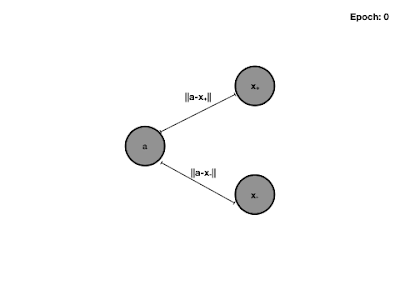## Sunday, July 7, 2019

### Implementing Triplet Loss Function in Tensorflow 2.0

In this post I will go through an implementation of the triplet loss for siamese neural network architectures in keras (tensorflow 2.0).

The goal of training a neural network with a triplet loss is to learn a metric embedding. That is examples that are conceptually close are also close in euclidean space and examples that are conceptually further are further away in euclidean space, too. The triplet loss is introduced in the facenet paper from google. When learning with the triplet loss, we choose three examples. The first example is called the anchor, which can be any example from our dataset. The positive example is one that is conceptually close. For example, a conceptually close example for face recognition might be a picture of the same person as shown in the anchor image. The negative example might simply be a picture of any other person. The embedding network can actually have any architecture. During training, we push all three examples through the network, compute the loss function with the three embeddings and adjust the weights which in each epoch pushes negative examples further from it's anchors and positive examples closer.Figure 1: The Triplet loss forces negative examples further from the anchor and positive examples closer.
An example is shown below. The loss consists of three parts. The first is the euclidean distance between the anchor and it's positive example. The second is the euclidean distance between the anchor and it's negative example. Since we want to minimize the loss, the first term should be small and the second term should be large. Furthermore, we introduce a regularization term or margin between the examples.Figure 2: We embed the anchor, positive and negative examples with the same network and apply the triplet loss. During Backpropagation we achieve higher euclidean distances to the negative example and lower distances for the negative examples.

For unsupervised audio embedding, one way to sample triplets is to pick a window from the audio as the anchor and a close window in time to the anchor as positive (since audio does not change that rapidly). The negative example is simply a sample from another file.

In order to implement such a loss in  Tensorflow 2.0 / Keras, we can implement the Loss base class.

class TripletLoss(loss.Loss):

def __init__(self, margin):
super().__init__()
self.margin = margin

def call(self, y_true, y_pred):
anchor = y_pred
pos    = y_pred
neg    = y_pred
pos_dist   = tf.reduce_sum(tf.square(tf.subtract(anchor, pos)), axis=-1)
neg_dist   = tf.reduce_sum(tf.square(tf.subtract(anchor, neg)), axis=-1)
loss       = tf.reduce_sum(tf.maximum(basic_loss, 0.0))
return loss


As you can see from the code, we do not need the ground truth (y truth) and we simply pass dummy values during training (Keras will still check it's dimension). In order to construct a model using the triplet loss, we can build an embedder model and then use that model three times in the triplet loss model. During back propagation, the three gradients will be summed and then passed through the embedder model (deep learning book chapter 6, Algorithm 6.6). In order to see a full example for
audio data, you can check this gist.